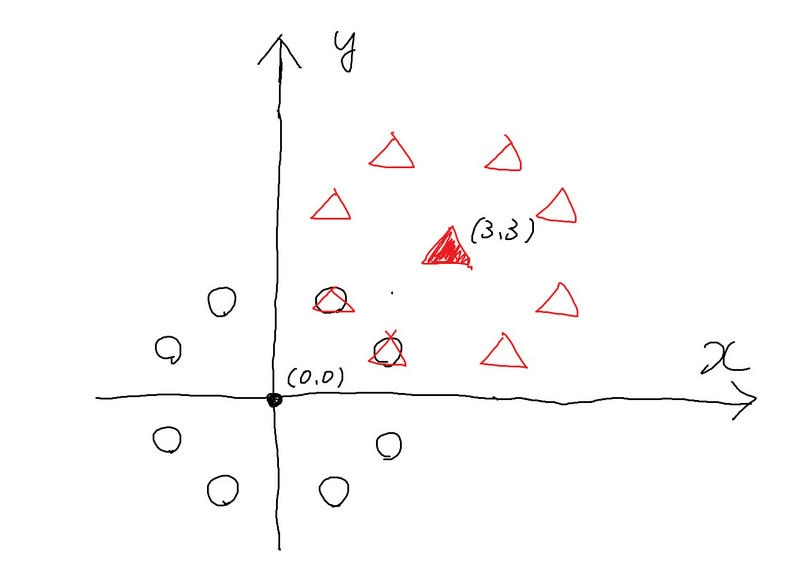Contest Duration: - (local time) (100 minutes) Back to Home

## C - Knight Fork Editorial by en_translator

The following is a naive brute-force implementation which enumerates $$(x, y)$$ from a wide range and checks if there is a point whose distances from $$(x_1, y_1)$$ and $$(x_2, y_2)$$ are both $$\sqrt{5}$$.

# Caclculates the squared distance
def dist_sq(a, b, c, d):
return (a - c) ** 2 + (b - d) ** 2

def solve(x1, y1, x2, y2):
for x in range(-2 * 10 ** 9, 2 * 10 ** 9 + 1):
for y in range(-2 * 10 ** 9, 2 * 10 ** 9 + 1):
if dist_sq(x, y, x1, y1) == dist_sq(x, y, x2, y2) == 5:
return "Yes"
return "No"


However, the computational complexity of this code is (the range of $$x$$) $$\times$$ (the range of $$y$$), which leads to TLE (Time Limit Exceeded).

It is important, not only in this problem, to draw an illustration when you are stuck in solving. Let’s draw.

For Sample 1, here is an illustration where the circle points denote $$(x_1, y_1)$$ and lattice points whose distances from $$(x_1, y_1)$$ is $$\sqrt{5}$$, and the triangle points denote $$(x_2, y_2)$$ and lattice points whose distances from $$(x_2, y_2)$$ is $$\sqrt{5}$$.From the illustration, we can see that the only points we have to inspect is “the points included in the $$4 \times 4$$ square centered at $$(x_1, y_1)$$.” This is because all the points outside the square is more distant than $$3$$, which exceeds $$\sqrt{5}$$.

The following is a program in which fewer points are inspected. This program is fast enough to get AC (Accepted).

# Caclculates the squared distance
def dist_sq(a, b, c, d):
return (a - c) ** 2 + (b - d) ** 2

def solve(x1, y1, x2, y2):
for x in range(x1 - 2, x1 + 3):
for y in range(y1 - 2, y1 + 3):
if dist_sq(x, y, x1, y1) == dist_sq(x, y, x2, y2) == 5:
return "Yes"
return "No"

x1, y1, x2, y2 = map(int, input().split())
print(solve(x1, y1, x2, y2))


posted:
last update: